Custom SearchVERNIER Sometimes the marking on the thimble of the micrometer does not fall directly on the index line of the sleeve. To make possible readings even smaller than thousandths, an ingenious device is introduced in the form of an additional scale. This scale, called a VERNIER, was named after its inventor, Pierre Vernier. The vernier makes possible accurate readings to the ten-thousandth of an inch. Principle of the Vernier Suppose a ruler has markings every tenth of an inch but it is desired to read accurately to hundredths. A separate scale (fig. 6-3) is added to the ruler. It has 10 markings on it that take up the same distance as 9 markings on the ruler scale. Thus, each space on the vernier is 1/10 of 9/10 inch, or 9/100 inch. How much smaller is a space on the vernier than a space on the ruler? The ruler space is 1/10 inch, or 10/100 and the vernier space is 9/100 inch. The vernier space is smaller by the difference between these two numbers, as follows: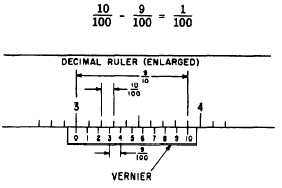Figure 6-3.-Vernier scale. Each vernier space is 1/100 inch smaller than a ruler space. As an example of the use of the vernier scale, suppose that we are measuring the steel bar shown in figure 6-4. The end of the bar almost reaches the 3-inch mark on the ruler, and we estimate that it is about halfway between 2.9 inches and 3.0 inches. The vernier marks help us to decide whether the exact measurement is 2.94 inches, 2.95 inches, or 2.96inches.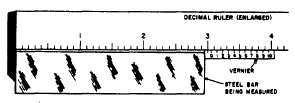Figure 6-4.-Measuring with a vernier, The 0 on the vernier scale is spaced the distance of exactly one ruler mark (in this case, one tenth- of an inch) from the left hand end of  the vernier. Therefore the 0 is at a position between ruler marks which is comparable to the position of the end of the bar. In other words, the 0 on the vernier is about halfway between two adjacent marks on the ruler, just as the end of the bar is about halfway between two adjacent marks. The 1 on the vernier scale is a little closer to alignment with an adjacent ruler mark; in fact, it is one hundredth of an inch closer to alignment than the 0. This is because each space on the vernier is one hundredth of an inch shorter than each space on the ruler. Each successive mark on the vernier scale is one hundredth of an inch closer to 0 on the vernier must be five hundredths of an inch from the nearest ruler mark, since five increments, each one hundredth of an inch in size, were used before a mark was found in alignment. We conclude that the end of the bar is five hundredths of an inch from the 2.9 mark on the ruler, since its position between marks is exactly comparable to that of the 0 on the vernier scale. Thus the value of our measurement is 2.95 inches. The foregoing example could be followed through for any distance between markings. Suppose the 0 mark fell seven tenths of the distance between ruler markings. It would take seven vernier markings, a loss of one-hundredth of an inch each time, to bring the marks in line at 7 on the vernier. The vernier principle may be used to get fine linear readings, angular readings, etc. The principle is always the same. The vernier has one more marking than the number of markings on an equal space of the conventional scale of the measuring instrument. For example, the vernier caliper (fig. 6-5) has 25 markings on the vernier for 24 on the caliper scale. The caliper is marked off to read to fortieths (0.025) of an inch, and the vernier extends the accuracy to a thousandth of an inch.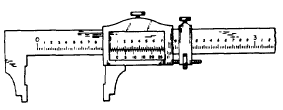Figure 6-5.–A vernier caliper. Vernier Micrometer By adding a vernier to the micrometer, it is possible to read accurately to one ten-thousandth of an inch. The vernier markings are on the sleeve of the micrometer and are parallel to the thimble markings. There are 10 divisions on the vernier that occupy the same space as 9 divisions on the thimble. Since a thimble space is one thousandth of an inch, a vernier space is 1/10 of 9/1000 inch, or 9/10000 inch. It is 1/10000 inch less than a thimble space. Thus, as in the preceding explanation of verniers, it is possible to read the nearest ten-thousandth of an inch by reading the vernier digit whose marking coincides with a thimble marking. In figure 6-6 (A), the last major division showing fully on the sleeve index is 3. The third minor division is the last mark clearly showing (0.075). The thimble division nearest and below the index is the 8 (0.008). The vernier marking that matches a thimble marking is the fourth (0.0004). Adding them all together, we have,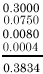The reading is 0.3834 inch. With practice these readings can be made directly from the micrometer, without writing the partial readings.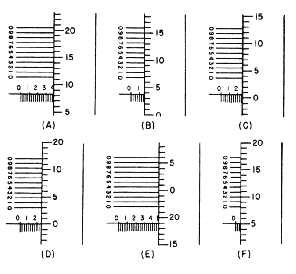Figure 6-6.–Vernier micrometer settings. Practice problems: 1. Read the micrometer settings in figure 6-6. Answers: 1. (A) See the foregoing example. (B) 0.1539  (C) 0.2507  (D) 0.2500 (E) 0.4690 (F) 0.0552Privacy Statement - Copyright Information. - Contact Us